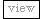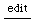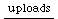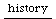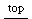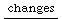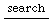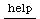## Random LFOs

just a few wrappers for LFNoise
mattr
 ```// smoothly varying between 0 and 1 RandLFO { *kr { arg freq = 1, mul = 1, add = 0; ^LFNoise2.kr(freq,0.5,0.5) * mul + add; } } // smoothly varying between -1 and 1 RandLFO2 { *kr { arg freq = 1, mul = 1, add = 0; ^LFNoise2.kr(freq) * mul + add; } } // between -1 and 1 in line segments // (use of LFNoise1 means that start point is random, unlike LFNoise2) RandLFO4 { *kr { arg freq = 1, mul = 1, add = 0; ^LFNoise1.kr(freq) * mul + add; } } // between 0 and 1 in line segments // (use of LFNoise1 means that start point is random, unlike LFNoise2) RandLFO5 { *kr { arg freq = 1, mul = 1, add = 0; ^(LFNoise1.kr(freq) * 0.5 * mul) + (0.5 * mul) + add; } } ``` /* authors so far: mattr */